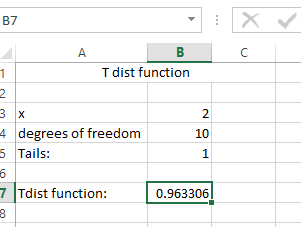### How To Calculate T Value In Excel

In this Article we will learn a very useful financial term know as T-Value.

T-Value is a statistical calculation using the mean, standard deviation and degrees of freedom and it will help in financial projections and forecasting.

With this information, a business owner can calculate the statistical low and high for earnings in a specific month and with a degree of confidence that the future earnings will fall within that range, which helps with better business planning. Projections can suggest that the business can expect to gross \$150,000 in May and the T-Value might tell them that number has a 90-percent rate of confidence. If the confidence rate is less than 50-percent, the business can plan for the potential of a lower return and work to increase the T-Value for future months and years.

Let us learn you how to calculate the T value using a simple formula in Excel:

The Formula

=T.DIST(x,deg_freedom,tails)

The T.DIST function uses the following arguments:

• X (required argument) – This is the numeric value at which we wish to evaluate the T Distribution.
• Deg_freedom (required argument) – An integer that indicates the number of degrees of freedom.
• Tails (required argument) – This specifies the number of distribution tails that would be returned. If tails =1, T.DIST returns the one-tailed distribution. If tails = 2, T.DIST returns the two-tailed distribution.

Lets create the data for the calculation:

T dist function

• x: 2
• degrees of freedom: 10
• Tails: 1

The formula is applied as below:

=T.DIST(B3,B4,B5)Result is:Tdist functions result is 0.963306

```Further reading:
How to calculate p value?```

## Template

```Further reading: